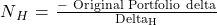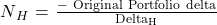Essential Concept 81: Delta Hedging and Gamma Risk | IFT World
101 Concepts for the Level I Exam

# Essential Concept 81: Delta Hedging and Gamma RiskDelta hedging refers to managing the portfolio delta by entering additional positions into the portfolio. If DeltaH is the delta of the hedging instrument, the optimal number of units of the hedging instruments, NH, is given by the formula below:Example: Suppose we know S = 100, X = 100, r = 5%, T = 1.0, σ = 30%, and δ = 5%. We have a short position in put options on 10,000 shares of stock. Based on this information, we note Deltac = 0.532, and Deltap = –0.419. Assume each stock option contract is for one share of stock.

1. Assuming the hedging instrument is a stock, how many stocks should be bought/sold for a delta hedge?
2. Assuming the hedging instrument is a call option, how many call options should be bought/sold for a delta hedge?

Solution to 1:

B is correct.The put delta is given as –0.419, thus the short put delta is 0.419. In this case, Portfolio delta = 10,000(0.419) = 4,190 and DeltaH = 1.0. Thus, the number of hedging units is –4,190 [= –(4,190/1)] or short sell 4,190 shares of stock.

Solution to 2:

A is correct. Again, the Portfolio delta = 4,190 but now DeltaH = 0.532. Thus, the number of hedging units is –7,875.9 [= –(4,190/0.532)] or sell 7,876 call options.

A few key points related to gamma are as follows:

• Gamma is always non-negative.
• Gamma has its largest value when an option is at the money.
• Gamma changes as the stock price changes and time to expiration change.
• Gamma approximates the estimation error in delta for options because the option price with respect to the stock is non-linear.
• Gamma measures the non-linearity risk or the risk of the delta neutral portfolio.
• A gamma neutral portfolio means gamma is zero.
• When hedging an option portfolio, the option gamma has to be managed and then the portfolio delta is neutralized.